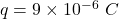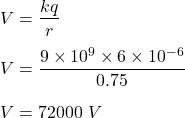calcula el potencial electrico de un punto B que se encuentra a 75cm de una varga positica q=9×10-6c​

Question

calcula el potencial electrico de un punto B que se encuentra a 75cm de una varga positica q=9×10-6c​

in progress 0
5 months 2021-08-12T23:26:21+00:00 1 Answers 3 views 0

V = 72000 volts

Explanation:

calculates the electric potential of a point B that is 75cm from a positive charge q = 9 × 10-6c

Given that,

Electric charge,We need to find the electric potential of a point B that is 75 cm from this charge. The formula for the electric potential is given by :So, the electric potential is equal to 72000 Volts.